Definition

The term adjusted total fertility rate or tempo-adjusted total fertility rate refers to a modified form of the total fertility rate that accounts for the tempo effect in a manner that provides (arguably) a better estimate of the completed fertility rate in the long term.

The standard definition of total fertility rate is that it is the sum of the age-specific fertility rates for all ages. Alternatively, it is the number of live births a hypothetical woman would have if she passed through her childbearing years conforming to the age-specific fertility rate of this particular year. If age-specific fertility rates hold constant over time, i.e., in the absence of tempo effects, the total fertility rate would equal the completed fertility rate for a birth cohort of females who have completed their childbearing years.

In the presence of tempo effects, we expect that age-specific fertility rates will change over time. The tempo-adjusted total fertility rate attempts to use data measuring the number, nature, and temporal distribution of live births in the current year and immediately surrounding years.

Bongaarts-Feeney formula

The formula works as follows. We will denote by$t$ the year for which we are attempting to estimate the tempo-adjusted total fertility rate. We assume that we have data for years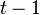$t - 1$ and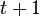$t + 1$. Further, we assume that the data we have is sufficient to compute not only the age-specific fertility rates, but the age-specific fertility rates broken down based on birth order.

We provide a hierarchical breakdown of the computation of the formula:

1. We compute the tempo-adjusted total fertility rate as the sum, over all possible birth orders, of the tempo-adjusted total fertility rate for that birth order.
2. For each birth order$i$, we compute the tempo-adjusted total fertility rate for that birth order as the product of the total fertility rate for that birth order and an adjustment factor.
1. The total fertility rate for that birth order is defined as the sum of the age-specific fertility rates for that birth order.
2. The adjustment factor is defined as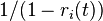$1/(1 - r_i(t))$ where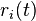$r_i(t)$ is the rate of change of mean age of childbearing for birth order$i$. Explicitly,$r_i(t)$ is estimated as the half-difference between the mean age of childbearing values for the next year and the previous year: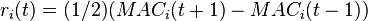$r_i(t) = (1/2)(MAC_i(t + 1) - MAC_i(t - 1))$. Here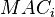$MAC_i$ denotes the mean age of childbearing at birth order$i$.

Overall, the formula is: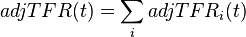$adjTFR(t) = \sum_i adjTFR_i(t)$

where: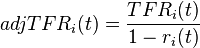$adjTFR_i(t) = \frac{TFR_i(t)}{1 - r_i(t)}$

where: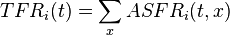$TFR_i(t) = \sum_x ASFR_i(t,x)$

(with the right side denoting summation over age-specific fertility rates at a given birth order)

and: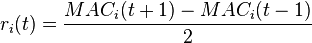$r_i(t) = \frac{MAC_i(t + 1) - MAC_i(t - 1)}{2}$

Values

Most recent values

Values here are based on the Human Fertility Database, a highly reliable source, but one that is limited to a few countries.